• +91 9971497814
• info@interviewmaterial.com

# Chapter 12 Introduction to Three Dimensional Geometry Ex-12.2 Interview Questions Answers

### Related Subjects

Question 1 :
Find the distance between the following pairs of points:
(i) (2, 3, 5) and (4, 3, 1) (ii) (–3, 7, 2) and (2, 4, –1)
(iii) (–1, 3, –4) and (1, –3, 4) (iv) (2, –1, 3) and (–2, 1, 3)

The distance between points P(x1, y1, z1) and P(x2, y2, z2) is given by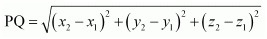(i) Distance between points (2, 3, 5) and (4, 3, 1)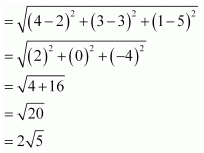(ii) Distance between points (–3, 7, 2) and (2, 4, –1)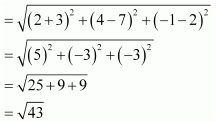(iii) Distance between points (–1, 3, –4) and (1, –3, 4)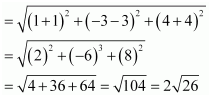(iv) Distance between points (2, –1, 3) and (–2, 1, 3)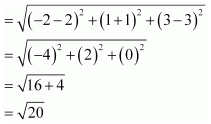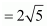Question 2 : Show that the points (–2, 3, 5), (1, 2, 3) and (7, 0, –1) are collinear.

Let points (–2, 3, 5), (1, 2, 3), and (7, 0, –1) be denoted by P, Q, and R respectively.
Points P, Q, and R are collinear if they lie on a line.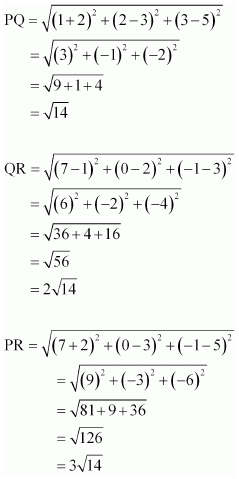Here, PQ + QR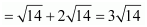= PR
Hence, points P(–2, 3, 5), Q(1, 2, 3), and R(7, 0, –1) are collinear.

Question 3 :
Verify the following:
(i) (0, 7, –10), (1, 6, –6) and (4, 9, –6) are the vertices of an isosceles triangle.
(ii) (0, 7, 10), (–1, 6, 6) and (–4, 9, 6) are the vertices of a right angled triangle.
(iii) (–1, 2, 1), (1, –2, 5), (4, –7, 8) and (2, –3, 4) are the vertices of a parallelogram.

(i) Let points (0, 7, –10), (1, 6, –6), and (4, 9, –6) be denoted by A, B, and C respectively.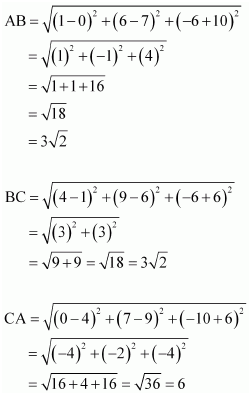Here, AB = BC ≠ CA
Thus, the given points are the vertices of an isosceles triangle.

(ii) Let (0, 7, 10), (–1, 6, 6), and (–4, 9, 6) be denoted by A, B, and C respectively.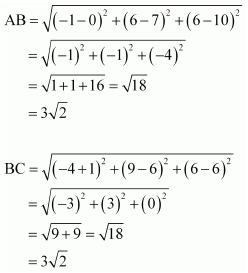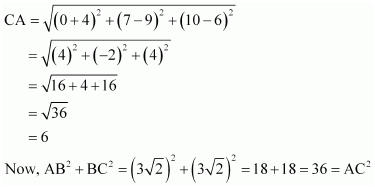Therefore, by Pythagoras theorem, ABC is a right triangle.
Hence, the given points are the vertices of a right-angled triangle.

(iii) Let (–1, 2, 1), (1, –2, 5), (4, –7, 8), and (2, –3, 4) be denoted by A, B, C, and D respectively.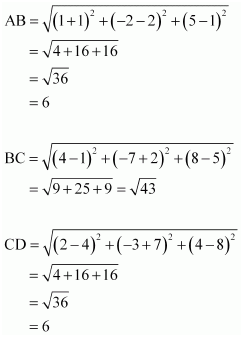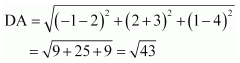Here, AB = CD = 6, BC = AD =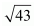Hence, the opposite sides of quadrilateral ABCD, whose vertices are taken in order, are equal.
Therefore, ABCD is a parallelogram.
Hence, the given points are the vertices of a parallelogram.

Question 4 : Find the equation of the set of points which are equidistant from the points (1, 2, 3) and (3, 2, –1).

Let P (xy, z)be the point that is equidistant from points A(1, 2, 3) and B(3, 2, –1).

Accordingly, PA = PB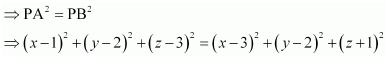x2 –2x + 1 + y2 – 4+ 4+ z2 – 6z + 9 = x2 –6x + 9 + y2 – 4y + 4+ z2 + 2z + 1

–2x –4y –6z + 14 = –6x – 4y + 2+ 14

– 2x – 6z +6x – 2z = 0

4x – 8z =0

x – 2z =0

Thus, the requiredequation is x – 2z = 0.

Question 5 : Find the equation of the set of points P, the sum of whose distances from A (4, 0, 0) and B (–4, 0, 0) is equal to 10.

Let the coordinates of P be (xyz).

The coordinates of points A and B are (4, 0, 0) and (–4, 0, 0) respectively.

It is given that PA + PB = 10.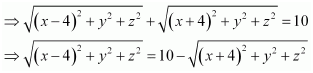Onsquaring both sides, we obtain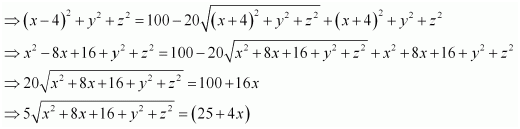Onsquaring both sides again, we obtain

25 (x2 +8+ 16 + y2 + z2) = 625 + 16x2 + 200x

25x2 + 200x +400 + 25y2 +25z2 =625 + 16x2 +200x

9x2 + 25y2 + 25z2 – 225 = 0

Thus, therequired equation is 9x2 + 25y2 + 25z2 – 225 = 0.

Todays Deals### Chapter 12 Introduction to Three Dimensional Geometry Ex-12.2 Contributorskrishan

Name:
Email:

# Latest News# 9000 interview questions in different categories